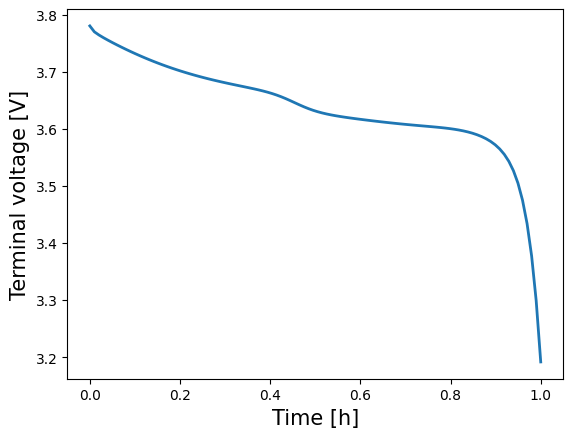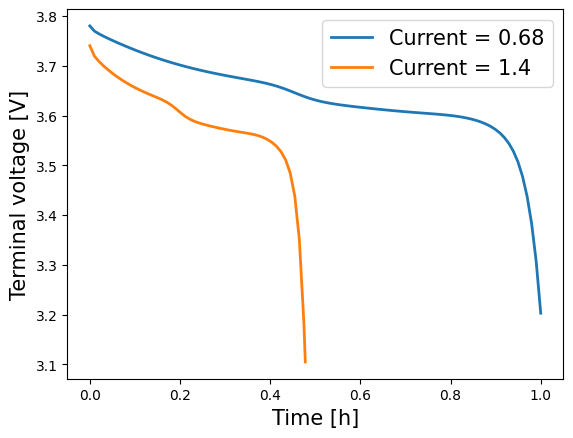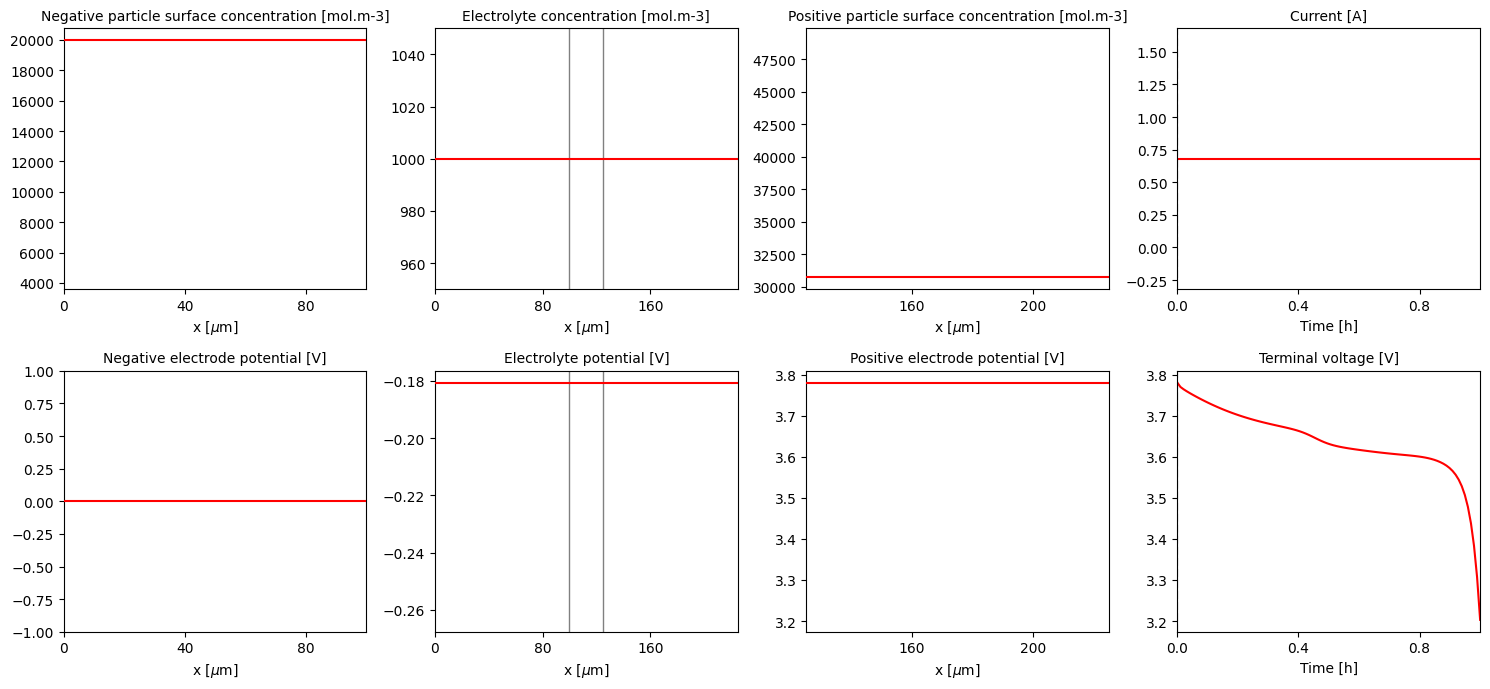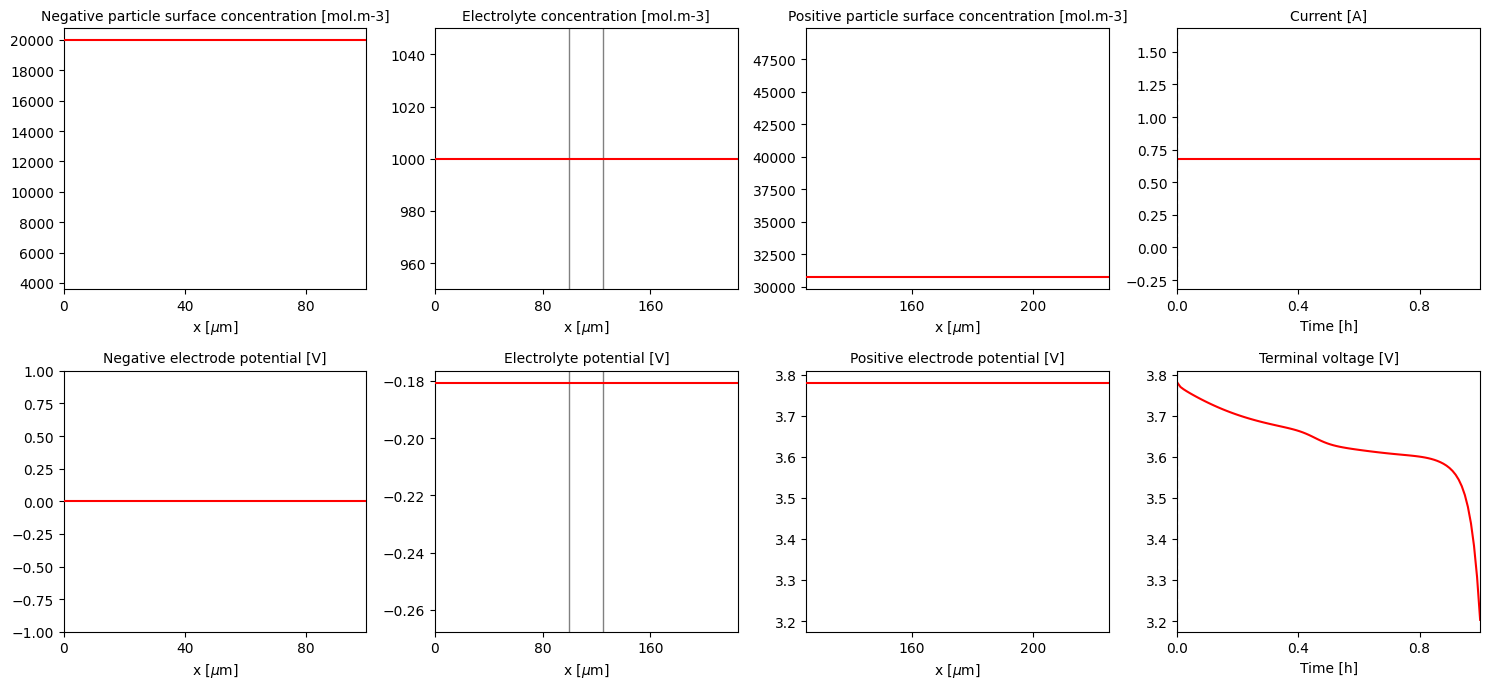Tip

An interactive online version of this notebook is available, which can be accessed via# Changing settings when solving PyBaMM models#

This example notebook showed how to run an SPM battery model, using the default parameters, discretisation and solvers that were defined for that particular model. Naturally we would like the ability to alter these options on a case by case basis, and this notebook gives an example of how to do this, again using the SPM model. In this notebook we explicitly handle all the stages of setting up, processing and solving the model in order to explain them in detail. However, it is often simpler in practice to use the Simulation class, which handles many of the stages automatically, as shown here.

1. Default SPM model

2. Changing the parameters

3. Changing the discretisation

4. Changing the solver

### The default SPM model#

Below is the code to define and run the default SPM model included in PyBaMM (if this is unfamiliar to you, please see this notebook for more details)

:

%pip install "pybamm[plot,cite]" -q    # install PyBaMM if it is not installed
import pybamm
import numpy as np
import os
import matplotlib.pyplot as plt
os.chdir(pybamm.__path__+'/..')

# create the model
model = pybamm.lithium_ion.SPM()

# set the default model geometry
geometry = model.default_geometry

# set the default model parameters
param = model.default_parameter_values

# set the parameters for the model and the geometry
param.process_model(model)
param.process_geometry(geometry)

# mesh the domains
mesh = pybamm.Mesh(geometry, model.default_submesh_types, model.default_var_pts)

# discretise the model equations
disc = pybamm.Discretisation(mesh, model.default_spatial_methods)
disc.process_model(model)

# Solve the model at the given time points
solver = model.default_solver
n = 100
t_eval = np.linspace(0, 3600, n)
solution = solver.solve(model, t_eval)

WARNING: You are using pip version 22.0.4; however, version 22.3.1 is available.
You should consider upgrading via the '/home/mrobins/git/PyBaMM/env/bin/python -m pip install --upgrade pip' command.
Note: you may need to restart the kernel to use updated packages.


To verify the solution, we can look at a plot of the Voltage over time

:

time = solution["Time [h]"].entries
voltage = solution["Voltage [V]"].entries
plt.plot(time, voltage, lw=2, label=model.name)
plt.xlabel("Time [h]", fontsize=15)
plt.ylabel("Voltage [V]", fontsize=15)
plt.show()### Changing the model parameters#

The parameters are defined using the pybamm.ParameterValues <https://docs.pybamm.org/en/latest/source/api/parameters/parameter_values.html>__ class, which takes either a python dictionary or CSV file with the mapping between parameter names and values.

First lets have a look at the default parameters that are included with the SPM model:

:

model.default_parameter_values.items

:

<bound method ParameterValues.items of {'Thermodynamic factor': 1.0,
'Ambient temperature [K]': 298.15,
'Bulk solvent concentration [mol.m-3]': 2636.0,
'Cation transference number': 0.4,
'Cell cooling surface area [m2]': 0.0569,
'Cell volume [m3]': 7.8e-06,
'Current function [A]': 0.680616,
'EC diffusivity [m2.s-1]': 2e-18,
'EC initial concentration in electrolyte [mol.m-3]': 4541.0,
'Edge heat transfer coefficient [W.m-2.K-1]': 0.3,
'Electrode height [m]': 0.137,
'Electrode width [m]': 0.207,
'Electrolyte conductivity [S.m-1]': <function electrolyte_conductivity_Capiglia1999 at 0x7fb36b261160>,
'Electrolyte diffusivity [m2.s-1]': <function electrolyte_diffusivity_Capiglia1999 at 0x7fb36b2611f0>,
'Initial concentration in electrolyte [mol.m-3]': 1000.0,
'Initial concentration in negative electrode [mol.m-3]': 19986.609595075,
'Initial concentration in positive electrode [mol.m-3]': 30730.7554385565,
'Initial inner SEI thickness [m]': 2.5e-09,
'Initial outer SEI thickness [m]': 2.5e-09,
'Initial temperature [K]': 298.15,
'Inner SEI electron conductivity [S.m-1]': 8.95e-14,
'Inner SEI lithium interstitial diffusivity [m2.s-1]': 1e-20,
'Inner SEI open-circuit potential [V]': 0.1,
'Inner SEI partial molar volume [m3.mol-1]': 9.585e-05,
'Inner SEI reaction proportion': 0.5,
'Lithium interstitial reference concentration [mol.m-3]': 15.0,
'Lower voltage cut-off [V]': 3.105,
'Maximum concentration in negative electrode [mol.m-3]': 24983.2619938437,
'Maximum concentration in positive electrode [mol.m-3]': 51217.9257309275,
'Negative current collector conductivity [S.m-1]': 59600000.0,
'Negative current collector density [kg.m-3]': 8954.0,
'Negative current collector specific heat capacity [J.kg-1.K-1]': 385.0,
'Negative current collector surface heat transfer coefficient [W.m-2.K-1]': 0.0,
'Negative current collector thermal conductivity [W.m-1.K-1]': 401.0,
'Negative current collector thickness [m]': 2.5e-05,
'Negative electrode Bruggeman coefficient (electrode)': 1.5,
'Negative electrode Bruggeman coefficient (electrolyte)': 1.5,
'Negative electrode OCP [V]': <function graphite_mcmb2528_ocp_Dualfoil1998 at 0x7fb36b261670>,
'Negative electrode OCP entropic change [V.K-1]': <function graphite_entropic_change_Moura2016 at 0x7fb36b261550>,
'Negative electrode active material volume fraction': 0.6,
'Negative electrode cation signed stoichiometry': -1.0,
'Negative electrode charge transfer coefficient': 0.5,
'Negative electrode conductivity [S.m-1]': 100.0,
'Negative electrode density [kg.m-3]': 1657.0,
'Negative electrode diffusivity [m2.s-1]': <function graphite_mcmb2528_diffusivity_Dualfoil1998 at 0x7fb36b2ad040>,
'Negative electrode double-layer capacity [F.m-2]': 0.2,
'Negative electrode electrons in reaction': 1.0,
'Negative electrode exchange-current density [A.m-2]': <function graphite_electrolyte_exchange_current_density_Dualfoil1998 at 0x7fb36b2615e0>,
'Negative electrode porosity': 0.3,
'Negative electrode reaction-driven LAM factor [m3.mol-1]': 0.0,
'Negative electrode specific heat capacity [J.kg-1.K-1]': 700.0,
'Negative electrode thermal conductivity [W.m-1.K-1]': 1.7,
'Negative electrode thickness [m]': 0.0001,
'Negative tab centre y-coordinate [m]': 0.06,
'Negative tab centre z-coordinate [m]': 0.137,
'Negative tab heat transfer coefficient [W.m-2.K-1]': 10.0,
'Negative tab width [m]': 0.04,
'Nominal cell capacity [A.h]': 0.680616,
'Number of cells connected in series to make a battery': 1.0,
'Number of electrodes connected in parallel to make a cell': 1.0,
'Outer SEI open-circuit potential [V]': 0.8,
'Outer SEI partial molar volume [m3.mol-1]': 9.585e-05,
'Outer SEI solvent diffusivity [m2.s-1]': 2.5000000000000002e-22,
'Positive current collector conductivity [S.m-1]': 35500000.0,
'Positive current collector density [kg.m-3]': 2707.0,
'Positive current collector specific heat capacity [J.kg-1.K-1]': 897.0,
'Positive current collector surface heat transfer coefficient [W.m-2.K-1]': 0.0,
'Positive current collector thermal conductivity [W.m-1.K-1]': 237.0,
'Positive current collector thickness [m]': 2.5e-05,
'Positive electrode Bruggeman coefficient (electrode)': 1.5,
'Positive electrode Bruggeman coefficient (electrolyte)': 1.5,
'Positive electrode OCP [V]': <function lico2_ocp_Dualfoil1998 at 0x7fb36b2613a0>,
'Positive electrode OCP entropic change [V.K-1]': <function lico2_entropic_change_Moura2016 at 0x7fb36b261280>,
'Positive electrode active material volume fraction': 0.5,
'Positive electrode cation signed stoichiometry': -1.0,
'Positive electrode charge transfer coefficient': 0.5,
'Positive electrode conductivity [S.m-1]': 10.0,
'Positive electrode density [kg.m-3]': 3262.0,
'Positive electrode diffusivity [m2.s-1]': <function lico2_diffusivity_Dualfoil1998 at 0x7fb36b2614c0>,
'Positive electrode double-layer capacity [F.m-2]': 0.2,
'Positive electrode electrons in reaction': 1.0,
'Positive electrode exchange-current density [A.m-2]': <function lico2_electrolyte_exchange_current_density_Dualfoil1998 at 0x7fb36b261310>,
'Positive electrode porosity': 0.3,
'Positive electrode reaction-driven LAM factor [m3.mol-1]': 0.0,
'Positive electrode specific heat capacity [J.kg-1.K-1]': 700.0,
'Positive electrode thermal conductivity [W.m-1.K-1]': 2.1,
'Positive electrode thickness [m]': 0.0001,
'Positive tab centre y-coordinate [m]': 0.147,
'Positive tab centre z-coordinate [m]': 0.137,
'Positive tab heat transfer coefficient [W.m-2.K-1]': 10.0,
'Positive tab width [m]': 0.04,
'Ratio of lithium moles to SEI moles': 2.0,
'Reference temperature [K]': 298.15,
'SEI growth activation energy [J.mol-1]': 0.0,
'SEI kinetic rate constant [m.s-1]': 1e-12,
'SEI open-circuit potential [V]': 0.4,
'SEI reaction exchange current density [A.m-2]': 1.5e-07,
'SEI resistivity [Ohm.m]': 200000.0,
'Separator Bruggeman coefficient (electrolyte)': 1.5,
'Separator density [kg.m-3]': 397.0,
'Separator porosity': 1.0,
'Separator specific heat capacity [J.kg-1.K-1]': 700.0,
'Separator thermal conductivity [W.m-1.K-1]': 0.16,
'Separator thickness [m]': 2.5e-05,
'Total heat transfer coefficient [W.m-2.K-1]': 10.0,
'Typical current [A]': 0.680616,
'Typical electrolyte concentration [mol.m-3]': 1000.0,
'Upper voltage cut-off [V]': 4.1,
'citations': ['Marquis2019']}>

:

format_str = '{:<75}  {:>20}'
print(format_str.format('PARAMETER', 'VALUE'))
print("-"*97)
for key, value in model.default_parameter_values.items():
try:
print(format_str.format(key, value))
except TypeError:
print(format_str.format(key, value.__str__()))

PARAMETER                                                                                   VALUE
-------------------------------------------------------------------------------------------------
Ratio of lithium moles to SEI moles                                                           2.0
Inner SEI reaction proportion                                                                 0.5
Inner SEI partial molar volume [m3.mol-1]                                               9.585e-05
Outer SEI partial molar volume [m3.mol-1]                                               9.585e-05
SEI reaction exchange current density [A.m-2]                                             1.5e-07
SEI resistivity [Ohm.m]                                                                  200000.0
Outer SEI solvent diffusivity [m2.s-1]                                       2.5000000000000002e-22
Bulk solvent concentration [mol.m-3]                                                       2636.0
Inner SEI open-circuit potential [V]                                                          0.1
Outer SEI open-circuit potential [V]                                                          0.8
Inner SEI electron conductivity [S.m-1]                                                  8.95e-14
Inner SEI lithium interstitial diffusivity [m2.s-1]                                         1e-20
Lithium interstitial reference concentration [mol.m-3]                                       15.0
Initial inner SEI thickness [m]                                                           2.5e-09
Initial outer SEI thickness [m]                                                           2.5e-09
EC initial concentration in electrolyte [mol.m-3]                                          4541.0
EC diffusivity [m2.s-1]                                                                     2e-18
SEI kinetic rate constant [m.s-1]                                                           1e-12
SEI open-circuit potential [V]                                                                0.4
SEI growth activation energy [J.mol-1]                                                        0.0
Negative electrode reaction-driven LAM factor [m3.mol-1]                                      0.0
Positive electrode reaction-driven LAM factor [m3.mol-1]                                      0.0
Negative current collector thickness [m]                                                  2.5e-05
Negative electrode thickness [m]                                                           0.0001
Separator thickness [m]                                                                   2.5e-05
Positive electrode thickness [m]                                                           0.0001
Positive current collector thickness [m]                                                  2.5e-05
Electrode height [m]                                                                        0.137
Electrode width [m]                                                                         0.207
Negative tab width [m]                                                                       0.04
Negative tab centre y-coordinate [m]                                                         0.06
Negative tab centre z-coordinate [m]                                                        0.137
Positive tab width [m]                                                                       0.04
Positive tab centre y-coordinate [m]                                                        0.147
Positive tab centre z-coordinate [m]                                                        0.137
Cell cooling surface area [m2]                                                             0.0569
Cell volume [m3]                                                                          7.8e-06
Negative current collector conductivity [S.m-1]                                        59600000.0
Positive current collector conductivity [S.m-1]                                        35500000.0
Negative current collector density [kg.m-3]                                                8954.0
Positive current collector density [kg.m-3]                                                2707.0
Negative current collector specific heat capacity [J.kg-1.K-1]                              385.0
Positive current collector specific heat capacity [J.kg-1.K-1]                              897.0
Negative current collector thermal conductivity [W.m-1.K-1]                                 401.0
Positive current collector thermal conductivity [W.m-1.K-1]                                 237.0
Nominal cell capacity [A.h]                                                              0.680616
Typical current [A]                                                                      0.680616
Current function [A]                                                                     0.680616
Negative electrode conductivity [S.m-1]                                                     100.0
Maximum concentration in negative electrode [mol.m-3]                            24983.2619938437
Negative electrode diffusivity [m2.s-1]                                      <function graphite_mcmb2528_diffusivity_Dualfoil1998 at 0x7fb36b2ad040>
Negative electrode OCP [V]                                                   <function graphite_mcmb2528_ocp_Dualfoil1998 at 0x7fb36b261670>
Negative electrode porosity                                                                   0.3
Negative electrode active material volume fraction                                            0.6
Negative electrode Bruggeman coefficient (electrolyte)                                        1.5
Negative electrode Bruggeman coefficient (electrode)                                          1.5
Negative electrode cation signed stoichiometry                                               -1.0
Negative electrode electrons in reaction                                                      1.0
Negative electrode charge transfer coefficient                                                0.5
Negative electrode double-layer capacity [F.m-2]                                              0.2
Negative electrode exchange-current density [A.m-2]                          <function graphite_electrolyte_exchange_current_density_Dualfoil1998 at 0x7fb36b2615e0>
Negative electrode density [kg.m-3]                                                        1657.0
Negative electrode specific heat capacity [J.kg-1.K-1]                                      700.0
Negative electrode thermal conductivity [W.m-1.K-1]                                           1.7
Negative electrode OCP entropic change [V.K-1]                               <function graphite_entropic_change_Moura2016 at 0x7fb36b261550>
Positive electrode conductivity [S.m-1]                                                      10.0
Maximum concentration in positive electrode [mol.m-3]                            51217.9257309275
Positive electrode diffusivity [m2.s-1]                                      <function lico2_diffusivity_Dualfoil1998 at 0x7fb36b2614c0>
Positive electrode OCP [V]                                                   <function lico2_ocp_Dualfoil1998 at 0x7fb36b2613a0>
Positive electrode porosity                                                                   0.3
Positive electrode active material volume fraction                                            0.5
Positive electrode Bruggeman coefficient (electrolyte)                                        1.5
Positive electrode Bruggeman coefficient (electrode)                                          1.5
Positive electrode cation signed stoichiometry                                               -1.0
Positive electrode electrons in reaction                                                      1.0
Positive electrode charge transfer coefficient                                                0.5
Positive electrode double-layer capacity [F.m-2]                                              0.2
Positive electrode exchange-current density [A.m-2]                          <function lico2_electrolyte_exchange_current_density_Dualfoil1998 at 0x7fb36b261310>
Positive electrode density [kg.m-3]                                                        3262.0
Positive electrode specific heat capacity [J.kg-1.K-1]                                      700.0
Positive electrode thermal conductivity [W.m-1.K-1]                                           2.1
Positive electrode OCP entropic change [V.K-1]                               <function lico2_entropic_change_Moura2016 at 0x7fb36b261280>
Separator porosity                                                                            1.0
Separator Bruggeman coefficient (electrolyte)                                                 1.5
Separator density [kg.m-3]                                                                  397.0
Separator specific heat capacity [J.kg-1.K-1]                                               700.0
Separator thermal conductivity [W.m-1.K-1]                                                   0.16
Typical electrolyte concentration [mol.m-3]                                                1000.0
Initial concentration in electrolyte [mol.m-3]                                             1000.0
Cation transference number                                                                    0.4
Thermodynamic factor                                                                                 1.0
Electrolyte diffusivity [m2.s-1]                                             <function electrolyte_diffusivity_Capiglia1999 at 0x7fb36b2611f0>
Electrolyte conductivity [S.m-1]                                             <function electrolyte_conductivity_Capiglia1999 at 0x7fb36b261160>
Reference temperature [K]                                                                  298.15
Ambient temperature [K]                                                                    298.15
Negative current collector surface heat transfer coefficient [W.m-2.K-1]                      0.0
Positive current collector surface heat transfer coefficient [W.m-2.K-1]                      0.0
Negative tab heat transfer coefficient [W.m-2.K-1]                                           10.0
Positive tab heat transfer coefficient [W.m-2.K-1]                                           10.0
Edge heat transfer coefficient [W.m-2.K-1]                                                    0.3
Total heat transfer coefficient [W.m-2.K-1]                                                  10.0
Number of electrodes connected in parallel to make a cell                                     1.0
Number of cells connected in series to make a battery                                         1.0
Lower voltage cut-off [V]                                                                   3.105
Upper voltage cut-off [V]                                                                     4.1
Initial concentration in negative electrode [mol.m-3]                             19986.609595075
Initial concentration in positive electrode [mol.m-3]                            30730.7554385565
Initial temperature [K]                                                                    298.15
citations                                                                         ['Marquis2019']


Most of the parameters in this list have numerical values. Some have string values, that point to particular python functions within PyBaMM. These denote parameters that vary over time and/or space, in a manner defined by the given python function. For the moment we will ignore these, and focus on altering one of the numerical parameters.

The class pybamm.ParameterValues <https://docs.pybamm.org/en/latest/source/api/parameters/parameter_values.html>__ acts like the normal python dict data structure, so you can read or write individual parameters accordingly:

:

variable = "Current function [A]"
old_value = param[variable]
param[variable] = 1.4
new_value = param[variable]
print(variable,'was',old_value)
print(variable,'now is',param[variable])

Current function [A] was 0.680616
Current function [A] now is 1.4


In order to compare solutions with different parameter values, parameters must be changed to InputParameter objects, whose value can then be specified when solving. For example, we can compare the Voltage calculated using both the old and new current values.

:

# Set up
model = pybamm.lithium_ion.SPM()
solver = model.default_solver
param["Current function [A]"] = "[input]"
param.process_model(model)
disc.process_model(model)

# Solution with current = 0.68
old_solution = solver.solve(model, t_eval, inputs={"Current function [A]": 0.68})
old_time = old_solution["Time [h]"].entries
old_voltage = old_solution["Voltage [V]"].entries

# Solution with current = 1.4
new_solution = solver.solve(model, t_eval, inputs={"Current function [A]": 1.4})
new_time = new_solution["Time [h]"].entries
new_voltage = new_solution["Voltage [V]"].entries

plt.plot(old_time, old_voltage, lw=2, label="Current = 0.68")
plt.plot(new_time, new_voltage, lw=2, label="Current = 1.4")
plt.xlabel("Time [h]", fontsize=15)
plt.ylabel("Voltage [V]", fontsize=15)
plt.legend(fontsize=15)
plt.show()Note that there is a small number of parameters for which this is not possible, since the discretisation depends on them (for example, Negative electrode thickness). In this case you would need to remesh the geometry as the relative length of the electrodes would have altered. This would in turn require you to re-discretise the model equations.

### Changing the discretisation#

The chosen spatial discretisation method to use for each domain is passed into the pybamm.Discretisation <https://docs.pybamm.org/en/latest/source/api/spatial_methods/discretisation.html>__ class as one of its arguments. The default spatial methods for the SPM class are given as:

:

print(format_str.format('DOMAIN', 'DISCRETISED BY'))
print("-"*82)
for key, value in model.default_spatial_methods.items():
print(format_str.format(key, value.__class__.__name__))

DOMAIN                                                                             DISCRETISED BY
----------------------------------------------------------------------------------
macroscale                                                                           FiniteVolume
negative particle                                                                    FiniteVolume
positive particle                                                                    FiniteVolume
negative primary particle                                                            FiniteVolume
positive primary particle                                                            FiniteVolume
negative secondary particle                                                          FiniteVolume
positive secondary particle                                                          FiniteVolume
negative particle size                                                               FiniteVolume
positive particle size                                                               FiniteVolume
current collector                                                            ZeroDimensionalSpatialMethod


To change the discretisation for a particular domain, you can update the spatial methods dict with the new discretiation class that you wish to use, for example:

:

spatial_methods = model.default_spatial_methods
spatial_methods["negative particle"] = pybamm.SpectralVolume()


You can also update the submeshes (meshes used for each domain) in a similar way. We’ll generate a spectral mesh to use with our spectral volume method in the negative particle

:

submesh_types = model.default_submesh_types
submesh_types["negative particle"] = pybamm.MeshGenerator(pybamm.SpectralVolume1DSubMesh)


Now that we have set the new discretisation method, we can proceed to re-discretise and then solve the model. Note that in this case we need to regenerate the model using pybamm.lithium_ion.SPM, as the original model information was lost when we discretised it above.

:

# re-generate the model and set parameters (with fixed current function this time)
model = pybamm.lithium_ion.SPM()
solver = model.default_solver
param["Current function [A]"] = 0.68
param.process_model(model)

# re-discretise the model with the new mesh...
mesh = pybamm.Mesh(geometry, submesh_types, model.default_var_pts)
disc = pybamm.Discretisation(mesh, spatial_methods)
disc.process_model(model)

# ... and finally solve it
solution = solver.solve(model, t_eval)
pybamm.QuickPlot(solution).plot(0)### Changing the solver#

Which method you use to integrate the discretised model in time can also be changed. PyBaMM has a number of different solvers available, all of which are described in the documentation.

:

print('Default solver for SPM model:',type(model.default_solver).__name__)

Default solver for SPM model: CasadiSolver


To change this, simply create a new solver using one of the available classes and use it to solve your discretised model

:

new_solver = pybamm.ScipySolver()
new_solution = new_solver.solve(model, t_eval)
pybamm.QuickPlot(new_solution).plot(0)